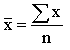### Mean monday (statistical interlude)In an effort to reimplement the somewhat abandoned concept ‘mean Monday,’ I’ve decided to take the mean testicle count of our contributors. For you lit-freaks that blow at math, the ‘mean’ or ‘arithmetic mean’ of any given set is “the sum of all of the list divided by the number of items in the list.” ‘Average’ is too broad and generic a term, as it may mean (no pun intended) ‘median’ or ‘mode.’Okay, here we go: there are 11 male contributors, all of whom (presumably) have two testicles, giving us a total of 22 testicles. There are 3 female contributors, giving us a total of 14 total contributors. Take 22 and divide by 14, and you have 1.57 — the number of testicles each contributor has. (Juxtapose this with 0.43 ovaries per contributor.)